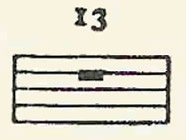A# rest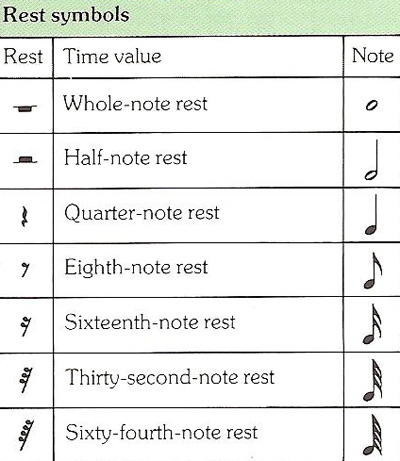Figure 1. Rest symbols.Figure 2. Eighth-note rests.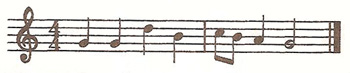Figure 3. An example of the use of one note or rest for several beats where several notes might have been used.Figure 4. Use of a two-beat rest.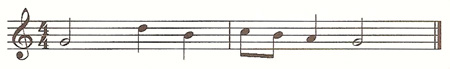Figure 5. Phrase beginning with a half-note.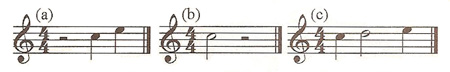Figure 6. Two-beat rests.

A rest is a direction not to play for a certain time period. It is designed to create silence in between separate notes or chords. For every note symbol, there is a rest symbol with an equal time value (Figure 1). So, like a note, a rest will tell you when to stop playing and for how long. In a bar of 4/4 time, a whole-note rest will mean that you stop playing for four beats, and a sixty-fourth note rest will mean that you stop for just a sixteenth of a beat. Clearly, the value of a rest symbol depends entirely on the time signature. The rest symbols are shown in the table. Note that the last four have the same number of flags on their stems as their equivalent note symbols.

## Examples of rests

In the example shown in Figure 2, in the first bar the time signature indicates four beats to the bar, each being a quarter-note (crotchet). Then come four single beats. In the second bar is a rest. This is an eighth-note (quaver) rest so it needs the value of half a beat to make up a quarter-note. This is done by making the first note in the bar an eighth-note. In the last bar, the two eighth-notes make the first quarter-note beat. Then comes an eighth-note followed by an eighth-note rest, making up the second beat. Finally the half-note (minim) makes up the last two beats.

Remember that the notes must be even and steady. Notice that, in order to fit a sound between the four numbered beats above, we have used an '&'. This '&' fits exactly between the two numbers. Imagine the ticking of a clock steadily fitting the numbers being counted.

It's also possible to use one note or rest for several beats where several notes might have been used. An example is shown in Figure 3.

If we wanted silence for beats three and four in the first bar we would write a two-beat rest and not two quarter-note rests, as shown in Figure 4.

If we wanted a single long note at the beginning we might write a half-note as in Figure 5.

We can generally write a two-beat rest (or note) at the beginning of a bar but rarely anywhere else. In 4/4 time, a two-beat rest can be on beats one and two, or three and four – but should not be written on beats two and three. Notes, however, may relax this rule.

In Figure 6, (a) and (b) are completely correct; (c) is, strictly speaking, grammatically incorrect – but this is a rhythmic pattern in constant and general use and, as such, the most appropriate way of notating this rhythm.

A silence of several bars is often indicated thus (or in some similar way):